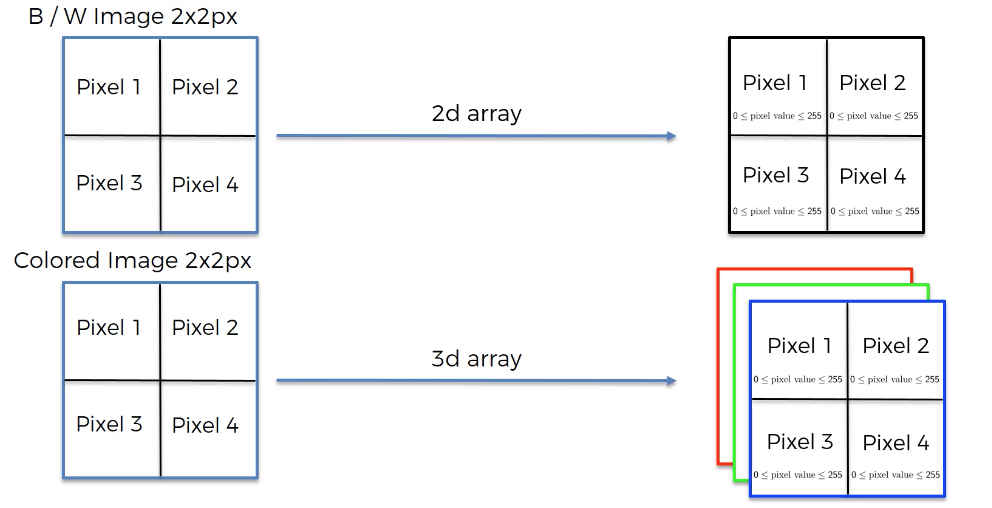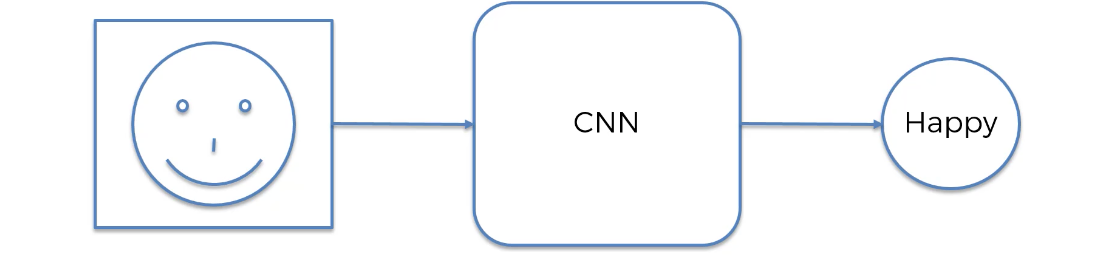# Introduction to Convolutional Neural Networks

Hey - Nick here! This page is a free excerpt from my \$199 course Python for Finance, which is 50% off for the next 50 students.

So far in this course, we have discussed deep learning specifically with respect to artificial neural networks (ANNs).

ANNs are an important and foundational predictive model used in deep learning. However, there are many other types of neural networks that are more useful in specific situations.

This article will introduce you to the next type of neural network we'll study in this course: convolutional neural networks.

## How Convolutional Neural Networks Are Used

Let's first discuss how convolutional neural networks are used.

In practice, the most common use case of convolutional neural networks is image identification. This means that convolutional neural networks can be used to identify objects within an image. Examples of this include:

• Recognizing stop signs from camera input for self-driving cars
• Recognizing animals in hunting cameras
• Generating meaningful search results for Google Images
• Tagging people in Facebook images

To really understand the importance and functionality of convolutional neural networks, you should have an understanding of their history. The next section

## The History of Convolutional Neural Networks

Convolutional neural networks were originally designed by Yann Lecun. You might say that Yann Lecun's influence in convolutional neural networks is similar to Geoffrey Hinton's influence in artificial neural networks.

In fact, Yann Lecun was actually a student under Geoffrey Hinton as a postdoctoral research associate. Hinton and Lecun - as well as fellow researcher Yoshua Bengio - are considered to be the most influential scientists in the field of deep learning. Lecun is now the Directory of Facebook AI Research (often abbreviated as FAIR) and is also a professor of NYU.

What makes convolutional neural networks different from the artificial neural networks that we have already discussed in this course is their structure. We will discuss the structure of convolutional neural networks next.

## The Structure of Artificial Neural Networks

At their most basic form, convolutional neural networks are used to classify images. For instance, here is a visual representation of how a convolutional neural network could be used to classify a person's emotion as `happy` in an image:The way that convolutional neural networks actually process these images is by transforming them into arrays. More specifically, images are transformed into two-dimensional arrays (also called matrices) where each entry in the array (or matrix) represents the color of the corresponding pixel.

In a black-and-whiet image, each pixel's color can have a value between `0` and `255`. This number corresponds to the grayscale value of the color of that specific pixel.

Color images are even more complex. Instead of being represented in a two-dimensional array, they are represented in a three-dimensional array where the third dimension is a dimension of length `3` for the basic `red`, `blue`, and `green` colors. Again, each element ranges from `0` to `255`, representing `256` bits of information.

Here is a visual representation of how convolutional neural networks interpret images:You'll learn more about how convolutional neural networks work as we proceed through this course. For now, it's enough to understand that images are deconstructed into arrays where the array contains values that correspond to the color of each pixel in the image.

## Problems With Convolutional Neural Networks

As with all statistical techniques, convolutional neural networks are not perfect. I wanted to present an example to conclude this article that demonstrates one situation in which a convolutional neural network will struggle.

Consider the following image:Do you see a duck or a rabbit?

Of course, there is no "right" answer. The illustration is designed to spur confusion. In fact, this image is historically significant because it is one of the earliest examples of an optical illusion.

It's also an excellent example of the type of image that would cause grief when analyzed by a convolutional neural network! Keep this example in mind as you move forward through this course, as it shows the fallibility of convolutional neural networks for image recognition.